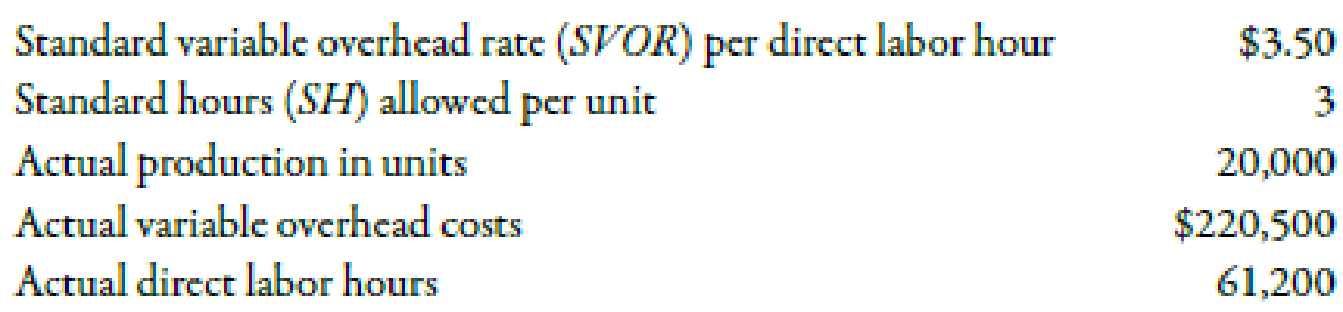Chapter 10, Problem 38BEB### Managerial Accounting: The Corners...

7th Edition
Maryanne M. Mowen + 2 others
ISBN: 9781337115773

#### Solutions

Chapter
Section### Managerial Accounting: The Corners...

7th Edition
Maryanne M. Mowen + 2 others
ISBN: 9781337115773
Textbook Problem
53 views

# Mulliner Company showed the following information for the year:Required: 1. Calculate the standard direct labor hours for actual production. 2. Calculate the applied variable overhead. 3. Calculate the total variable overhead variance.

1.

To determine

Compute the standard direct labor hours for the actual production.

Explanation

Standard Labor Hours Allowed:

The labor hours which are required to produce the actual amount of production output is known as standard hours allowed.

Use the following formula to calculate the amount of standard labor hours:

Standard Hours=Unit Labor Standard×Actual Output

Substitute 3 hours for unit labor standard and 20,000 for actual output in the above formula

2.

To determine

Compute the amount of applied variable overhead.

3.

To determine

Compute the amount of total variable overhead variance.

### Still sussing out bartleby?

Check out a sample textbook solution.

See a sample solution

#### The Solution to Your Study Problems

Bartleby provides explanations to thousands of textbook problems written by our experts, many with advanced degrees!

Get Started